#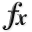N-D Test Functions B¶

class go_benchmark.BartelsConn(dimensions=2)

Bartels-Conn test objective function.

This class defines the Bartels-Conn global optimization problem. This is a multimodal minimization problem defined as follows: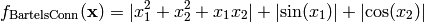Here,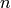represents the number of dimensions andfor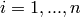.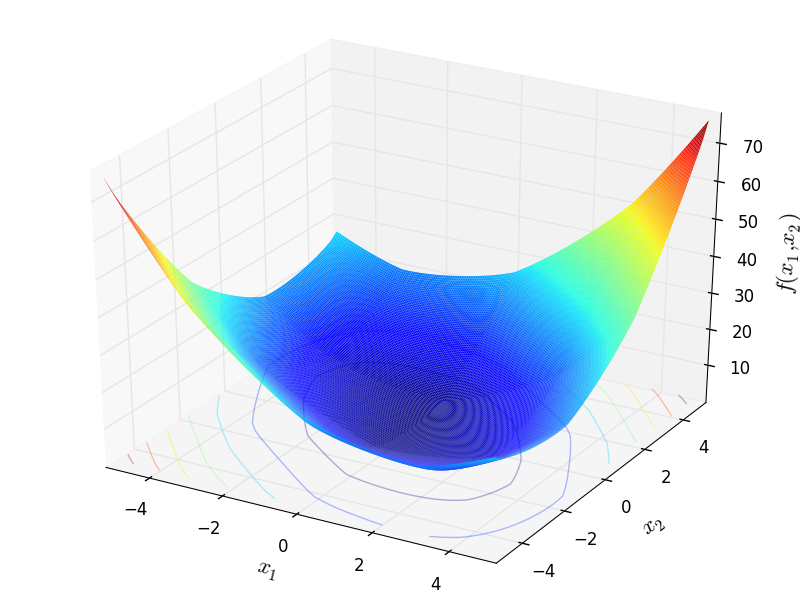Two-dimensional Bartels-Conn function

Global optimum: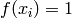for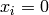forclass go_benchmark.Beale(dimensions=2)

Beale test objective function.

This class defines the Beale global optimization problem. This is a multimodal minimization problem defined as follows: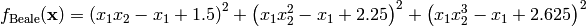Here,represents the number of dimensions andfor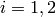.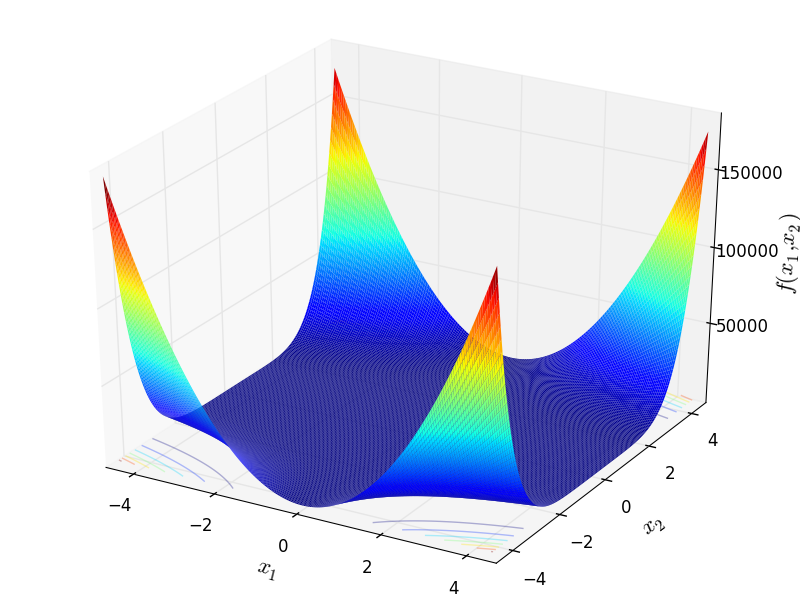Two-dimensional Beale function

Global optimum: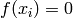for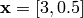class go_benchmark.Bird(dimensions=2)

Bird test objective function.

This class defines the Bird global optimization problem. This is a multimodal minimization problem defined as follows:Here,represents the number of dimensions and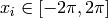for.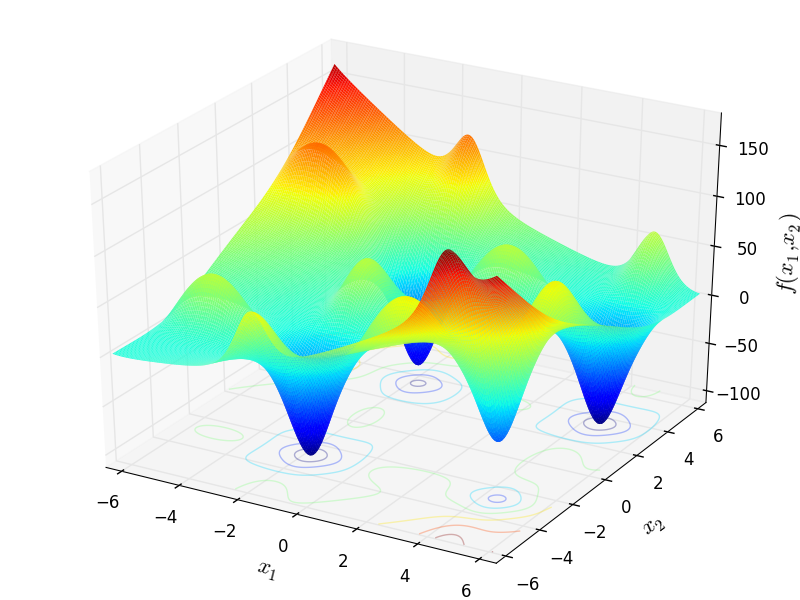Two-dimensional Bird function

Global optimum:for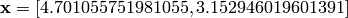or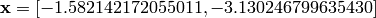class go_benchmark.Bohachevsky(dimensions=2)

Bohachevsky test objective function.

This class defines the Bohachevsky global optimization problem. This is a multimodal minimization problem defined as follows: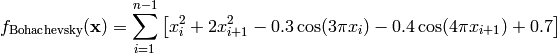Here,represents the number of dimensions and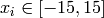for.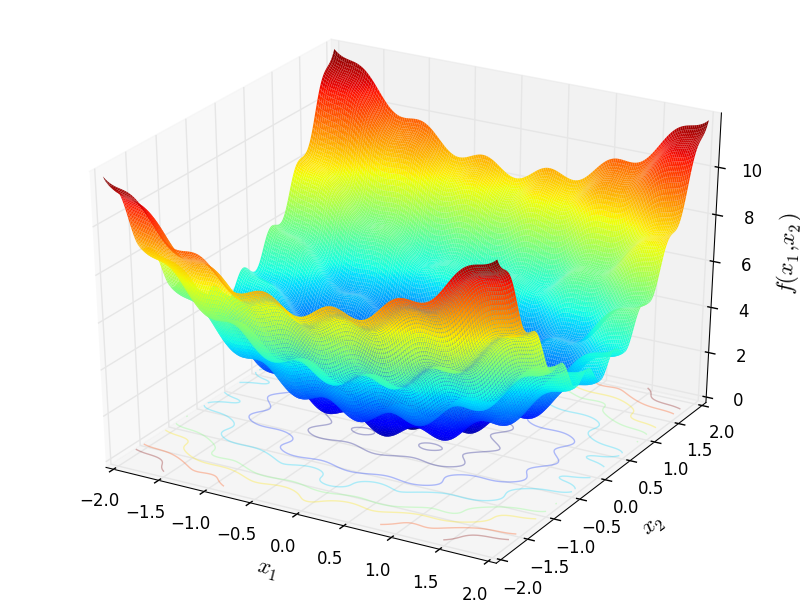Two-dimensional Bohachevsky function

Global optimum:forforclass go_benchmark.BoxBetts(dimensions=3)

BoxBetts test objective function.

This class defines the Box-Betts global optimization problem. This is a multimodal minimization problem defined as follows: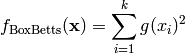Where, in this exercise: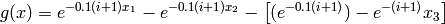And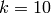.

Here,represents the number of dimensions and.

Global optimum:for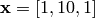class go_benchmark.Branin01(dimensions=2)

Branin 1 test objective function.

This class defines the Branin 1 global optimization problem. This is a multimodal minimization problem defined as follows: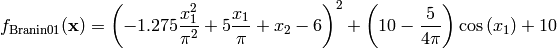Here,represents the number of dimensions and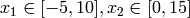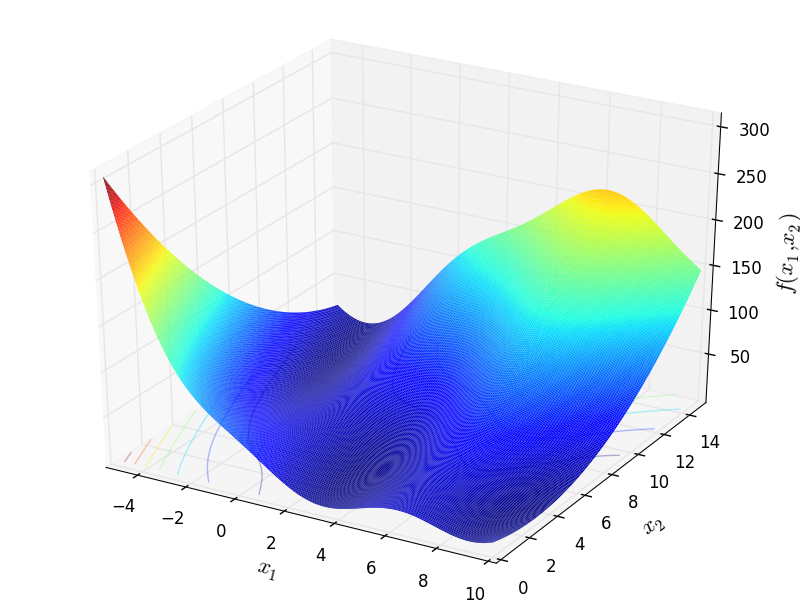Two-dimensional Branin 1 function

Global optimum: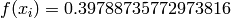for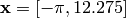or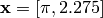or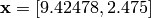class go_benchmark.Branin02(dimensions=2)

Branin 2 test objective function.

This class defines the Branin 2 global optimization problem. This is a multimodal minimization problem defined as follows: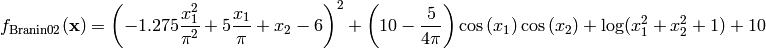Here,represents the number of dimensions andfor.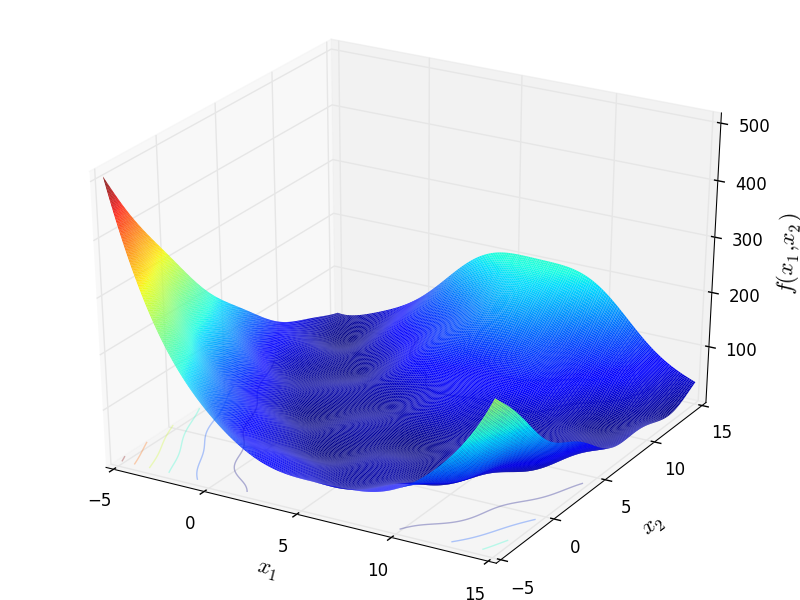Two-dimensional Branin 2 function

Global optimum: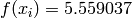for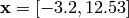class go_benchmark.Brent(dimensions=2)

Brent test objective function.

This class defines the Brent global optimization problem. This is a multimodal minimization problem defined as follows: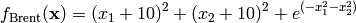Here,represents the number of dimensions andfor.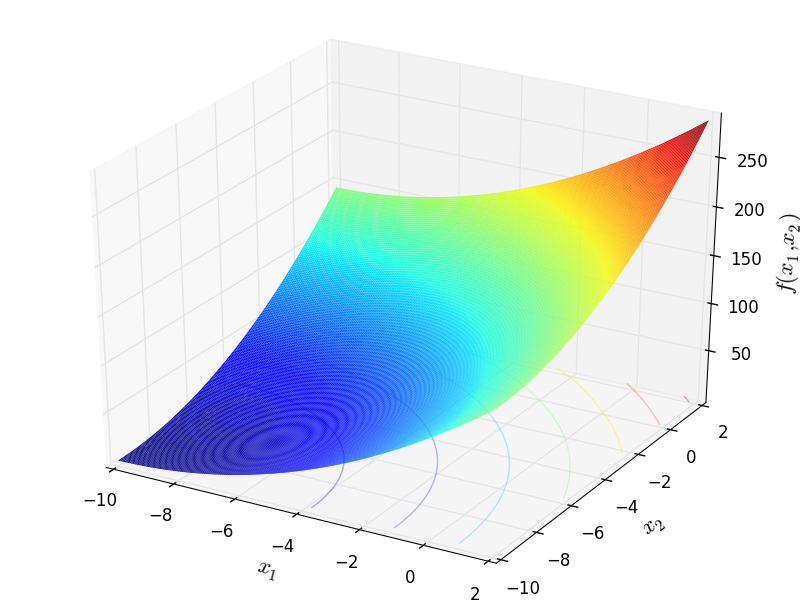Two-dimensional Brent function

Global optimum:for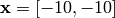class go_benchmark.Brown(dimensions=2)

Brown test objective function.

This class defines the Brown global optimization problem. This is a multimodal minimization problem defined as follows:Here,represents the number of dimensions and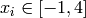for.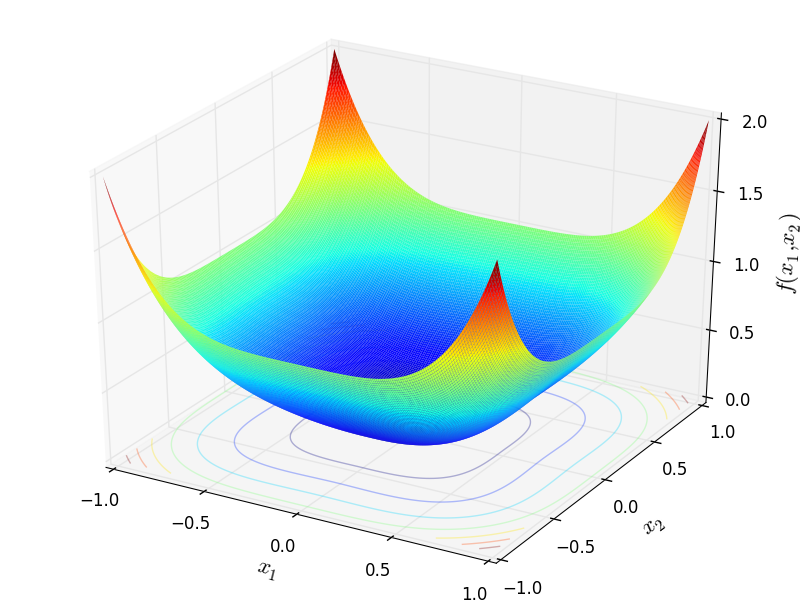Two-dimensional Brown function

Global optimum:forforclass go_benchmark.Bukin02(dimensions=2)

Bukin 2 test objective function.

This class defines the Bukin 2 global optimization problem. This is a multimodal minimization problem defined as follows: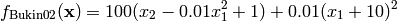Here,represents the number of dimensions and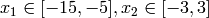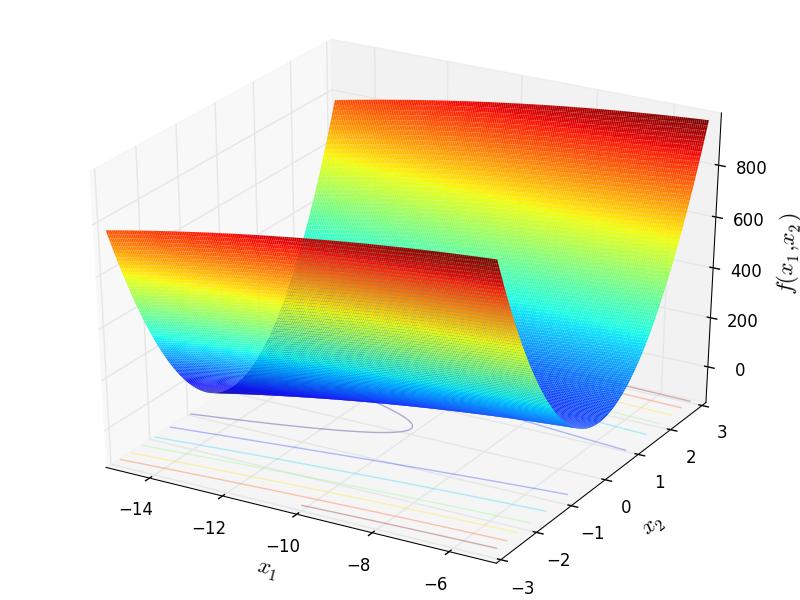Two-dimensional Bukin 2 function

Global optimum:forclass go_benchmark.Bukin04(dimensions=2)

Bukin 4 test objective function.

This class defines the Bukin 4 global optimization problem. This is a multimodal minimization problem defined as follows: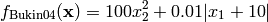Here,represents the number of dimensions and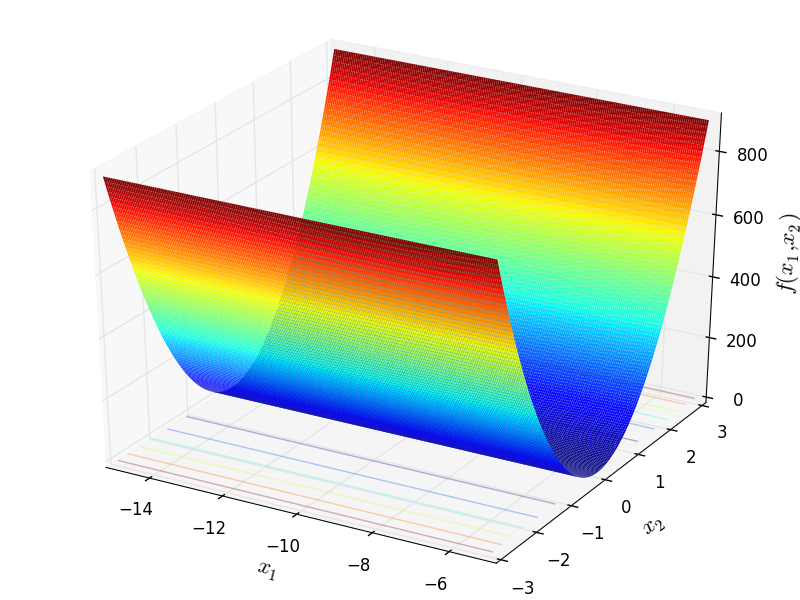Two-dimensional Bukin 4 function

Global optimum:forclass go_benchmark.Bukin06(dimensions=2)

Bukin 6 test objective function.

This class defines the Bukin 6 global optimization problem. This is a multimodal minimization problem defined as follows: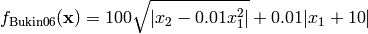Here,represents the number of dimensions and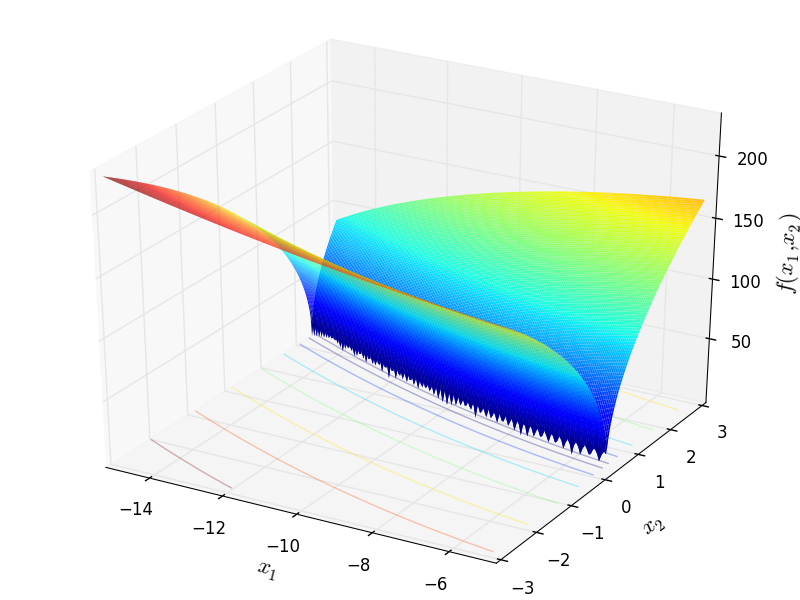Two-dimensional Bukin 6 function

Global optimum:for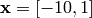#### Previous topic

N-D Test Functions A

#### Next topic

N-D Test Functions C